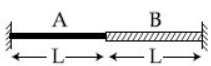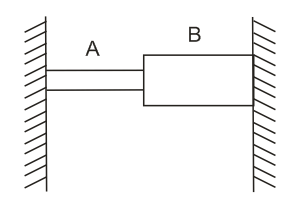#A wire of length is made by joining two wires A and B of same length but different radii r and 2r and made of the same material. It is vibrating at a frequency such that the joint of the two wires forms a node. If the number of antinodes in wire A is p and that in B is q then the ratio p:q is : Option 1) Option 2) Option 3)   Option 4)

S solutionqcSo

Option 1)

Option 2)

Option 3)

Option 4)

Exams
Articles
Questions